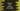# Python Program to find the factorial of a Number## Python program to find the factorial of a number using recursion :

The factorial of a number is the product of all the numbers from 1 to that number. e.g. factorial of 5 is 1 * 2 * 3 * 4 * 5 i.e. 120 . In this tutorial, we will learn how to find out the factorial of a number using a recursive method.

Factorial is denoted by “!”: 5 factorial is denoted by 5!

## Recursive method :

The recursive method calls itself to solve a problem. This is called a recursion process. These types of methods will call itself again and again till a certain condition is satisfied.

Finding out the factorial of a number is one of the classic problem used for recursion.

The factorial of a number ’n’ is the product of all numbers from ‘1’ to ‘n’. Or, we can say that the factorial of ’n’ is equal to ’n’ times the factorial of n - 1. If the value of ’n’ is ’1’, its factorial is ‘1’.

``````def fact(x):
if x == 0 :
return 1
return x * fact(x - 1)

print(fact(5))``````

code

We can implement it in python like below :

1. fact() method is used to find out the factorial of a number. It takes one number as its argument. The return value of this method is the factorial of the argument number. This method calls itself recursively to find out the factorial of the argument number.
2. Inside this method, we are checking if the value of the argument is ’1’ or not. If it is ’1’, we are returning ’1’. Else, we are returning the multiplication of the argument number to fact(x -1) or the factorial of the number* (x - 1)*. This line calls the same method again.
3. fact(x -1) will again call the method fact(). If the value of (x-1) is ’1’, it will return ’1’. Else, it will return (x -1) * fact(x -2) . So, the same method will be called again and again recursively.
4. This product chain will continue until the value of ’x’ is ’1’. It will return ‘x * (x - 1) * (x - 2)…1’ or the factorial of ’x‘.

The output of the above program is “120

## Explanation :

In the above example,

1. fact() function takes one argument “x“
2. If “x ” is “1“, it will return 1. Because we don’t need to find the factorial of* ‘1’. The factorial of ‘1’ is ‘1’* itself.
3. Else it will return x * fact(x-1) i.e. fact(x-1) will call fact() function one more time with* (x-1)* as argument . If ’x _’ is _10, it will call _fact(9). _
4. It will continue till x is 1, i.e. the function will return 1 and no more steps we need to move inside.

So, for 5,

1. it will call 5 * fact (4)
2. fact(4 ) will be 4 * fact (3)
3. fact(3) will be 3 * fact (2 )
4. fact(2) will be 2 * fact (1)
5. fact(1) will be 1
6. That means, the final output is

`5 * fact(4)`

`= 5 * 4 * fact(3)`

`= 5 * 4 * 3 * fact(2)`

`= 5 * 4 * 3 * 2 * fact(1)`

`= 5 * 4 * 3 * 2 * 1 * fact(0)`

`= 5 * 4 * 3 * 2 * 1 * 1`

`= 120`

Try changing the input number to different and check the result.

## Conclusion :

In this example, we have learned how to find the factorial of a number in python recursively. The recursive method comes in handy if you need to execute the same process again and again. Try to run the above example and try it with different numbers to find the factorial. You can download the program from the GitHub link mentioned above.

If you have any queries, don’t hesitate to drop one comment below.

__ View on Github